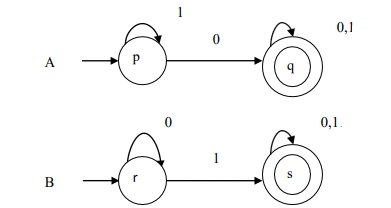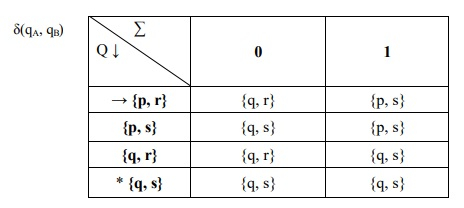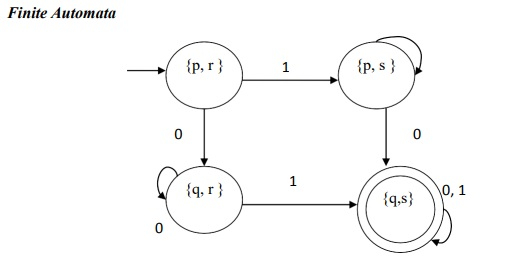# Explain the intersection process of two DFA’s

According to the theorem, If L and M are two regular languages, then L ∩ M is also regular language.

## Example

Construct A∩B where A and B is given as follows −The language A ={10,100,00,001,1010,…..}

The language B ={01,1010,10,101,…..}

AA = (QA, Σ, δA, qa, FA)
AB = (QB, Σ, δB, qB, FB)
A∩B=(QA x QB ,Σ,δ(qA x qB ,FA x F B )

Where,

δ(( p, q), a) =δL (p, a), δM (q, a))

Here, QA x QB = {p,q} x {r,s}
={(p, r), (p, s), (q, r), (q, s)}
Z = {0, 1}
qA x qB = {p, r}
FA x FB = {q, s}The finite automaton is given below −